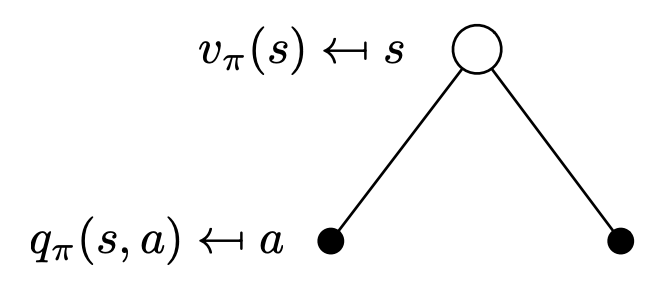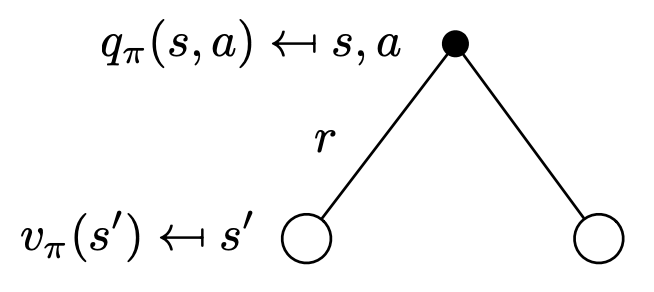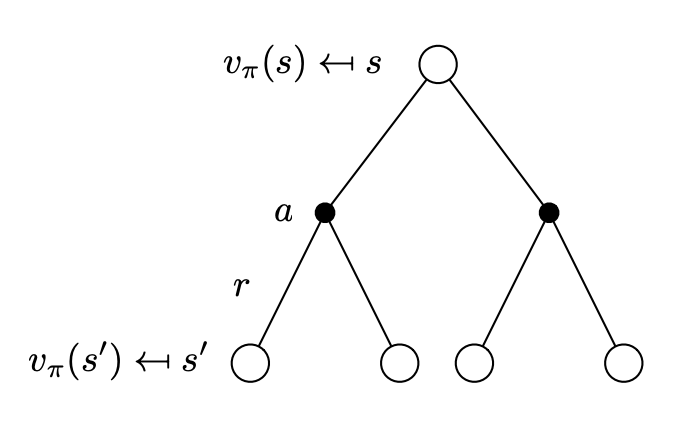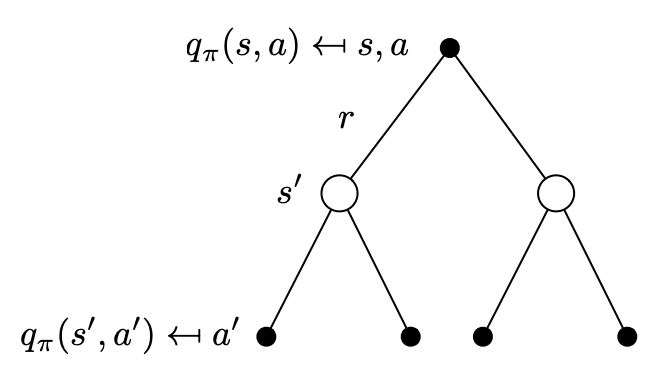# 贝尔曼方程

# 动作值函数的贝尔曼方程

$Q_{\pi}(s_t, a_t) = \mathbb{E}_{S_{t+1}, A_{t+1}} \Big [ R_t + \gamma \cdot Q_{\pi}(S_{t+1}, A_{t+1}) \mid S_t = s_t, A_t = a_t \Big ]$

$G_t = \sum_{k=0} \gamma^k R_{t+k} = R_t + \gamma \cdot G_{t+1}$

\begin{aligned} Q_{\pi}(s_t, a_t) &= \mathbb{E}_{S_{t+1}, A_{t+1}, \cdots} \Big [ G_t \mid S_t = s_t, A_t = a_t \Big ] \\ &= \mathbb{E}_{S_{t+1}, A_{t+1}, \cdots} \Big [ R_t + \gamma \cdot G_{t+1} \mid S_t = s_t, A_t = a_t \Big ] \\ &= \mathbb{E}_{S_{t+1}, A_{t+1}, \cdots} \Big [ R_t + \gamma \cdot Q_{\pi}(S_{t+1}, A_{t+1}) \mid S_t = s_t, A_t = a_t \Big ] \end{aligned}

# 状态值函数的贝尔曼方程

$V_{\pi}(s_t) = \mathbb{E}_{A_t, S_{t+1}} \Big [ R_t + \gamma \cdot V_{\pi}(S_{t+1}) \mid S_t = s_t \Big ]$

\begin{aligned} Q_{\pi}(S_t, A_t) &= \mathbb{E}_{S_{t+1}, A_{t+1}} \Big [ R_t + \gamma \cdot Q_{\pi}(S_{t+1}, A_{t+1}) \mid S_t = s_t, A_t = a_t \Big ] \\ &= \mathbb{E}_{S_{t+1}} \Big [ R_t + \gamma \cdot V_{\pi}(S_{t+1}) \mid S_t = s_t, A_t = a_t \Big ] \end{aligned}

\begin{aligned} V_{\pi}(S_t) &= \mathbb{E}_{A_t} \Big [ Q_{\pi}(S_t, A_t) \Big ] \\ &= \mathbb{E}_{A_t, S_{t+1}} \Big [ R_t + \gamma \cdot V_{\pi}(S_{t+1}) \mid S_t = s_t \Big ] \end{aligned}

# 另一种形式$V_{\pi}(s) = \sum_{a \in \mathcal{A}} \pi(a \mid s) Q_{\pi}(s, a) \tag{1}$$Q_{\pi}(s, a) = R(s, a) + \gamma \sum_{s' \in \mathcal{S}} P_{ss'}^a V_{\pi}(s') \tag{2}$$V_{\pi}(s) = \sum_{a \in \mathcal{A}} \pi(a \mid s) \Big( R(s, a) + \gamma \sum_{s' \in \mathcal{S}} P_{ss'}^a V_{\pi}(s') \Big) \tag{3}$$Q_{\pi}(s, a) = R(s, a) + \gamma \sum_{s' \in \mathcal{S}} P_{ss'}^a \sum_{a \in \mathcal{A}} \pi(a' \mid s') Q_{\pi}(s', a') \tag{4}$

# 贝尔曼最优方程

$V_*(s) = \sum_{a \in \mathcal{A}} \pi(a \mid s) Q_*(s, a)$

$Q_*(s, a) = R(s, a) + \gamma \sum_{s' \in \mathcal{S}} P_{ss'}^a V_*(s')$

$V_*(s) = \sum_{a \in \mathcal{A}} \pi(a \mid s) \Big( R(s, a) + \gamma \sum_{s' \in \mathcal{S}} P_{ss'}^a V_*(s') \Big) \tag{5}$

$Q_*(s, a) = R(s, a) + \gamma \sum_{s' \in \mathcal{S}} P_{ss'}^a \sum_{a \in \mathcal{A}} \pi(a' \mid s') Q_*(s', a') \tag{6}$